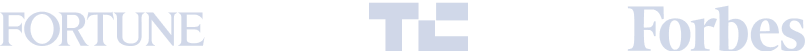# Free Math Resources

### Our instructors and team are working hard to make math learning accessible for families during this time. We’ll update this page weekly with freePre-Algebra and Algebra worksheets, video tutorials,andmore. Need help? Looking up your questions is one of the best ways to learn! Another great way to learn is from a Math instructor.Learn moreabout our Math curriculum and Math classes with live instructors.# Pre-Algebra

### Pre-Algebra is a middle school level course that prepares students for studying Algebra 1.

#### Pre-Algebra A

A covers four broad topics: arithmetic review, fractions, decimals, percents and ratios, arithmetic expressions, and exponents and radicals.

#### Pre-Algebra B

B follows directly after Pre-Algebra A. It covers four broad topics: data and statistics, geometry, number theory, and probability and counting.

Feel ready for the next level? Try some Algebra exercises below.

# Algebra

### Strengthen your algebra foundation by practicing linear and quadratic equations, and problem-solving skills.

#### Algebra 1A

• Algebra 1A covers three broad topics: solving linear equations, graphing linear equations, and solving systems of linear equations.

#### Algebra 1B

• Algebra 1B follows directly after Algebra 1A. It covers four broad topics: manipulating polynomials, solving quadratic equations, graphing quadratic equations, and functions.

#### Activities

• Scratch is perfect for introducing kids ages 8-11 to basic coding concepts, problem-solving, and logical thinking. Start learning with this beginning Scratch project tutorial.

# Keep Learning

### Find more tips, advice, and general information on Math and STEM.